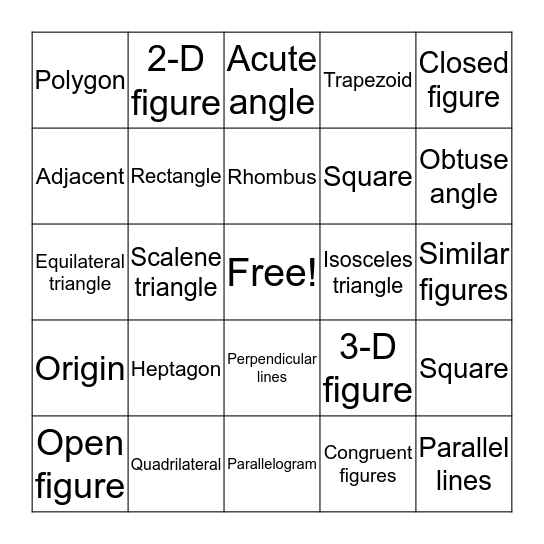# Geometry BingoThis bingo card has a free space and 24 words: Heptagon, Quadrilateral, Rhombus, Open figure, Closed figure, Polygon, Adjacent, 2-D figure, Isosceles triangle, Perpendicular lines, Square, Equilateral triangle, 3-D figure, Square, Rectangle, Congruent figures, Similar figures, Acute angle, Obtuse angle, Scalene triangle, Parallel lines, Origin, Trapezoid and Parallelogram.

⚠ This card has duplicate items: Square (2)

## Play Online